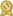Found 178 differential equations tutors in Brooklyn, NY 11201 – page 2 of 18.

New York 10001 · 4 miles from 11201 · \$495/hour · teaches Differential Equations

...differential equations, mathematical logic and set theory. I am also currently a math professor at Hofstra University, where I teach precalculus, multivariable calculus, differentialcertified

New York 10009 · 2 miles from 11201 · \$120/hour · teaches Precalculus

ACT Math - 36 SAT Math II - 790 AP BC Calculus - 5 Courses Taken at The Cooper Union: Calc I, Calc II, Vector Calc (Calc III), Differential Equations, Probability & Statistics, and Linear Algebra.certified

New York 10009 · 2 miles from 11201 · \$80/hour · teaches Algebra 1 - Calculus - Physics

My coursework at the Cooper Union has included up to Calculus 4 (differential equations) as well as additional classes in probability and linear algebra.certified

Jersey City 07306 · 5 miles from 11201 · \$100/hour · teaches Algebra 1 - Algebra 2 -

2) Rutgers University, Mathematics 2) Rutgers University, Graduate Coursework A certified tutor for mathematics. I have tutored in college various courses like Algebra, Calculus, Differential...certified

New York 10036 · 4 miles from 11201 · \$100/hour · teaches Differential Equations

McGill University, Electrical Eng University of Oxford, PhD I'm a dedicated and experienced math and science tutor who's had the privilege of teaching diverse and bright students at the...certified

New York 10016 · 4 miles from 11201 · \$50/hour · teaches Differential Equations

SUNY Potsdam, Mathematics SUNY Potsdam, Masters I am an inquisitive student of the seemingly disparate disciplines of mathematics and music. I am currently a Pure Mathematics PhD student at...certified

Brooklyn 11230 · 5 miles from downtown · \$35/hour · teaches Algebra 1 - Algebra 2 -

I have taught College Algebra, Trigonometry, PreCalculus, Calculus, and currently also teaching Differential equations, Plus I've been tutoring different ranges of ages from 10 to 50.certified

New York 10028 · 6 miles from 11201 · \$45/hour · teaches Algebra 1 - Algebra 2 -

My most knowledgeable fields are Physics, Calculus, Pre-calculus (including Trigonometry, Geometry, and Algebra), Differential Equations, and Linear Algebra. I enjoy working with all ages of students...certified

New York 10023 · 6 miles from 11201 · \$109/hour · teaches Differential

New Jersey Institute of Technology (NJIT), Applied Mathematics When I became a college student, I was offered a position at my University's math tutoring center. Because of my previous experiences as a tutor...certified

New York 10031 · 10 miles from 11201 · \$70/hour · teaches Algebra 1 - Algebra 2 -

SUBJECTS Precalculus, Differential Calculus, Integrable Calculus (AP Calc as well), Multivariable Calculus, Linear Algebra and Differential Equations, Complex Variables and Advance Linear Algebra...certified